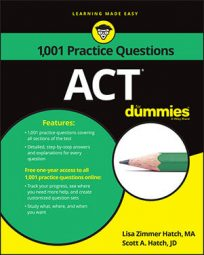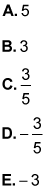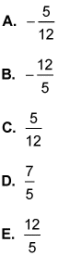##### ACTIf you want to hang out on the coordinate plane, then you should know how to use linear equations. On the ACT Math exam, you'll encounter questions like the following, where you're asked to break out the slope-intercept form to calculate the slopes of different lines.

## Practice questions

1. What is the slope of the line given by the equation 3x = 30 – 5y?2. In the standard xy-coordinate plane, what is the slope of the line that is perpendicular to the line 5y – 12x = 35?1. The correct answer is Choice (D).

Order the equation in slope-intercept form: y = mx + b. The m value will be the slope.

2. The correct answer is Choice (A).

To find the slope of the given line, put it in the slope-intercept form:

So the slope of the given line is 12/5.

To determine the slope of a perpendicular line, flip the fraction and change the sign. The slope has to be negative, so eliminate Choices (C), (D), and (E). Flip the numerator and denominator to get